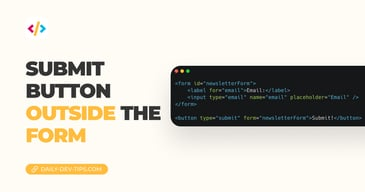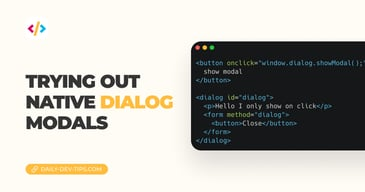Subscribe

# HTML output element

••••••• + 7 devs liked it

✍️

Defining the output for inputs in HTML

11 Dec, 2020 · 2 min read

I've seen this element around a couple of times in articles but never needed it or decided to see what it can do.

In general, it's supposed to be used as an output for other input elements.

Sounds pretty cool for certain use-cases.

Let's say we need to build a VAT calculator?

The end result:## Using the HTML output element permalink

To use this element we can simply write `<output name="output"></output>`.

But that doesn't mean it actually does anything.

To make it work, it needs a form wrapped around it and some inputs to mix values from.

Let's start by doing that.

``````<form
onsubmit="return false"
oninput="output.value = parseInt(inputOne.value) + parseInt(inputTwo.value)"
>
<input name="inputOne" type="number" value="2" /> +
<input name="inputTwo" type="number" value="3" /> =
<output name="output">5</output>
</form>``````

You might think, oh well, but that's cheating you just put some values in there.

The magic comes in running this code. Try and change the input values!

See the Pen HTML output element by Chris Bongers (@rebelchris) on CodePen.

As you can see it does rely on JavaScript to actually render a new value in the output element. In this case we state that `output.value` (the name output) equals a parsed one + two.

Note: Their names reference the elements used.

## HTML output VAT calculator permalink

Let's say we need an VAT calculator, these general have an input number and a vat percentage.

``````<form
onsubmit="return false"
oninput="output.value = price.valueAsNumber + (price.valueAsNumber * vat.valueAsNumber)"
>
<input name="price" type="number" value="20" /> +
<input name="vat" type="number" value="0.2" step="0.1" max="1" /> =
<output name="output">24</output>
</form>``````

As you can see we are no longer using the `parseInt` method. Instead we can use the `valueAsNumber` on input types. This makes it easier to use decimals.

Then we define the sum function as follows:

`output = price + (price * vat)`

The names above are all the names used in our HTML.

As you can see, not fancy scripts were needed, and we can fully style this any way we would like!

I hope you learned some good practical use cases for the HTML output element.

Feel free to have a play with this Codepen.

See the Pen HTML output element ~ VAT by Chris Bongers (@rebelchris) on CodePen.

This output element is pretty well supported and goes back to pretty old versions. We do run into issues on IE and Opera mini.Tweet this tip
••••••• + 7 devs liked it

### Submit button outside the form

4 Dec, 2022 · 1 min read### Trying out native dialog modals

8 Aug, 2022 · 3 min readJoin 2101 devs and subscribe to my newsletter

• 1000 articles written
• 2101 devs subscribed
• 529116 words written